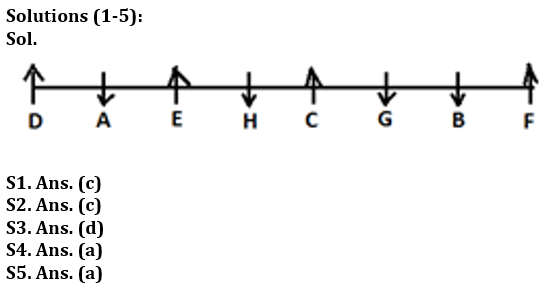Latest Banking jobs   »

# Reasoning Ability Quiz For LIC ADO Prelims 2023- 9th March

Directions (1-5): Study the following information carefully and answer the questions given below:
Eight persons A, B, C, D, E, F, G and H are sitting in a row. Four of them are facing towards north while four of them are facing towards south. No two person sits adjacent to each other according to the English alphabet i.e. (B does not sits adjacent to A and C).
C sits third to the left of A. Only two person sits between C and F. A does not sits at the end of the row. E sits 4th to the right of B. H sits third to the right of D and faces south. G and H faces same direction. Immediate neighbour of H faces same direction. F faces north direction.

Q1. Who among the following pair are facing same direction?
(a) All of them are facing same direction
(b) A, D
(c) A, B
(d) D, H
(e) A, F

Q2. Who among the following person sits immediate right of A?
(a) G
(b) A
(c) D
(d) No one
(e) B

Q3. What is the position of H with respect to F?
(a) Fourth to the right
(b) Immediate left
(c) Second to the right
(d) Fourth to the left
(e) Second to the left

Q4. How many persons are sitting between G and D?
(a) Four
(b) Two
(c) No one
(d) Three
(e) Six

Q5. Who among the following person are immediate neighbors of B?
(a) F, G
(b) A, F
(c) F, H
(d) D, C
(e) None of these

Direction (6-10): In these questions, relationship between different elements is show in the statements. The statements are followed by conclusions. Study the conclusions based on the given statements and select the appropriate answer:
(a) If only conclusion I follows.
(b) If only conclusion II follows.
(c) If either conclusion I or II follows
(d) If neither conclusion I nor II follows.
(e) If both conclusions I and II follow.

Q6. Statements: W=E>F>V, M≥W, G≥S≥V
Conclusion: I: E≤M II: M>S

Q7. Statements: H≤R, T<U≤H, H<I<R
Conclusion: I: I≥T II: I>T

Q8. Statements: R>S, P>M≥O, R=Q<O
Conclusion: I: M> R II: S<O

Q9. Statements: B<E<R<T, U≥Y=T, U=X
Conclusion: I: B>Y II: X>E

Q10. Statements: S<M<U, Y>X>R, T≥R, Y<U
Conclusion: I: X>M II: M≥X

Q11. How many pairs of letters are there in the word “ADEQUATE” each of which have as many letters between them in the word as they have between them in the English alphabetical series?
(a) Three
(b) One
(c) Two
(d) More than three
(e) None

Q12. If in the number 63143214, addition of 1 after multiplying by 2 in each of the odd digit and subtract 3 after multiplying by 2 in each of the even digit then, how many digits are repeating in new number?
(a) Three
(b) One
(c) None
(d) Two
(e) Four

Q13. Which of the following elements should come in a place of ‘?’
JQ4 HS9 FU16 ?
(a) EV36
(b) PK 81
(c) IR 100
(d) DW25
(e) LO 49

Q14. Arun’s ranked 38th from the top and 44th from the bottom in a class. How many students are there in the class?
(a) 82
(b) 77
(c) 81
(d) 79
(e) None of these

Q15. If in the number 867351429, all digits are arranged in increasing order from right to left than how many digit remain same place in the new arrangement?
(a) Three
(b) Four
(c) none
(d) Two
(e) One

SolutionsSolutions (6-10):
S6. Ans. (a)
Sol. I: E≤M(true) II: M>S(False)
S7. Ans. (b)
Sol. I: I≥T (False) II: I>T (True)
S8. Ans. (e)
Sol. I: M>R (True) II: S<O (True)
S9. Ans. (b)
Sol. I: B>Y (False) II: X>E (True)
S10. Ans. (c)
Sol. I: X>M (False) II: M≥X (False)

S11. Ans. (c)
Sol. (de, qt)

S12. Ans. (a)
Sol. Original number- 63143214
Obtained number- 97357135

S13. Ans. (d)

S14. Ans. (c)
Sol. Number of students in the class= 38+44-1=81

S15. Ans. (a)## FAQs

### When will the LIC AAO Prelims Exam 2023 be conducted?

The LIC AAO Prelims Exam 2023 will be conducted on 17th & 20th February 2023.

#### Congratulations!Union Budget 2023-24: Free PDF